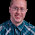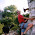## Tuesday, January 29, 2013

### java regular expression on byte array

Ever wanted to use a regular expresson on a byte array in Java? It turns out that regular expressions are eight bit safe in Java, and bytes can safely map into the lower half of the character type. With a simple adapter it becomes a trivial task. Demonstration:
```package org.yi.happy.binary_regex;

import static org.junit.Assert.assertEquals;

import java.util.regex.Matcher;
import java.util.regex.Pattern;

import org.junit.Test;

public class BinaryRegexTest {
/**
* Find line endings in a byte array using a regular expression.
*/
@Test
public void testExpression() {
byte[] data = new byte[] { 'a', '\r', '\r', 'c' };
Pattern p = Pattern.compile("\r\n?|\n\r?");
Matcher m = p.matcher(new ByteCharSequence(data));

assertEquals(true, m.find(0));
assertEquals(1, m.start());
assertEquals(2, m.end());

assertEquals(true, m.find(2));
assertEquals(2, m.start());
assertEquals(3, m.end());

assertEquals(false, m.find(3));
}

/**
* Find null bytes in a byte array using a regular expression.
*/
@Test
public void testNull() {
byte[] data = new byte[] { 'a', 0, 'b', 0 };

Pattern p = Pattern.compile("\0");
Matcher m = p.matcher(new ByteCharSequence(data));

assertEquals(true, m.find(0));
assertEquals(1, m.start());
assertEquals(2, m.end());

assertEquals(true, m.find(2));
assertEquals(3, m.start());
assertEquals(4, m.end());

assertEquals(false, m.find(4));
}
}
```
And the adapter is as one might expect,
```package org.yi.happy.binary_regex;

public class ByteCharSequence implements CharSequence {

private final byte[] data;
private final int length;
private final int offset;

public ByteCharSequence(byte[] data) {
this(data, 0, data.length);
}

public ByteCharSequence(byte[] data, int offset, int length) {
this.data = data;
this.offset = offset;
this.length = length;
}

@Override
public int length() {
return this.length;
}

@Override
public char charAt(int index) {
return (char) (data[offset + index] & 0xff);
}

@Override
public CharSequence subSequence(int start, int end) {
return new ByteCharSequence(data, offset + start, end - start);
}

}
```

1.This is brilliant! I can now do binary pattern matching using built-in java libs. The only thing I might suggest is to emphasise that in the expression \XYZ is in Oct (not hex, not dec).

Mate, thanks for sharing this!

2.Thanks Sarah, this was really helpful.

3.This is a helpful demo, but why is it necessary to "and" the byte with 0xff in the charAt() method? isn't casting the result to char enough?

4.Reading from byte[] should only returns 8 bits of data. So you would think "and" is not needed, however it is signed. Casting to 16 bit char and then using "and" makes sure the value is always positive.

"The char data type is a single 16-bit Unicode character. It has a minimum value of '\u0000' (or 0) and a maximum value of '\uffff' (or 65,535 inclusive)."
"The byte data type is an 8-bit signed two's complement integer. It has a minimum value of -128 and a maximum value of 127 (inclusive)."
https://docs.oracle.com/javase/tutorial/java/nutsandbolts/datatypes.html

You only need to "and" if you have byte values above 127 (which are two's complement negative values). But the code should always "and" to be safe.

Example java:

byte[] ba = {(byte)0xff};
char c1 = (char) ba ;
char c2 = (char) (ba & 0xff);
System.out.println((int)c1);
System.out.println((int)c2);

Output:
65535
255

5.